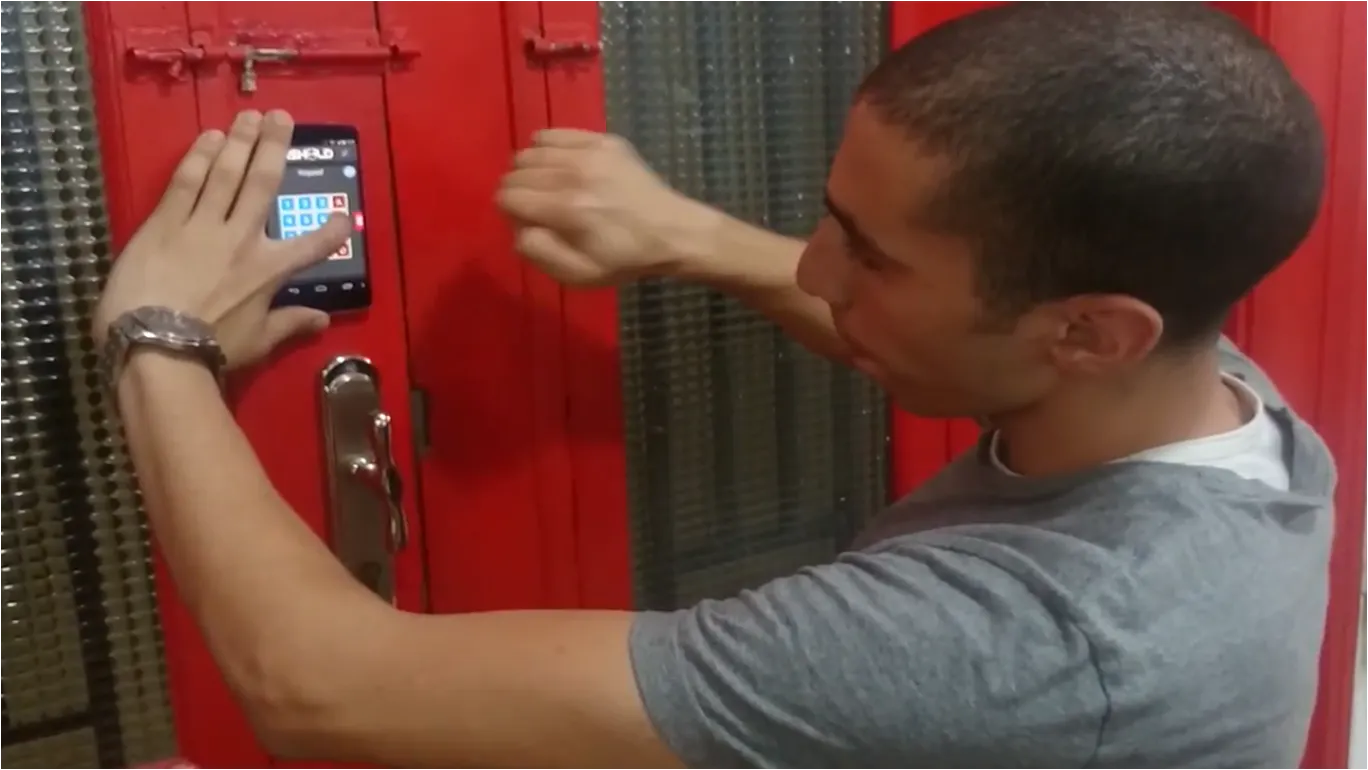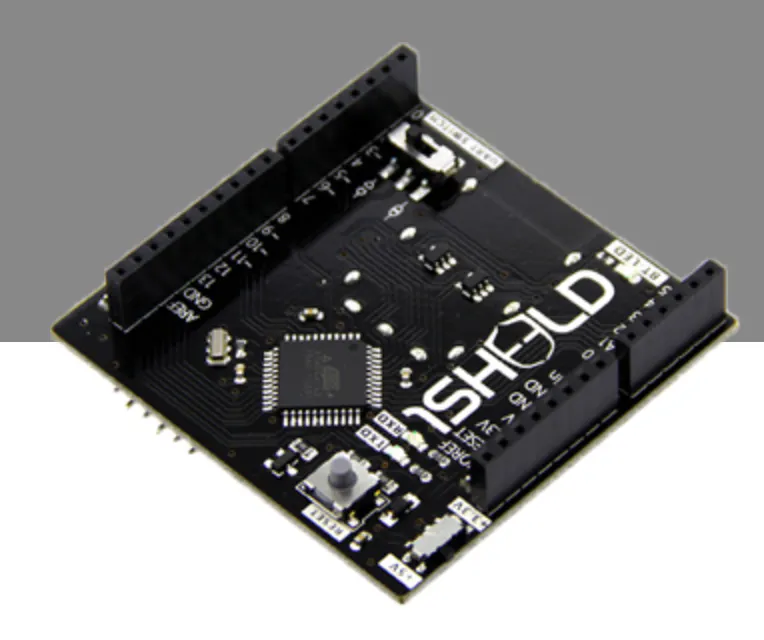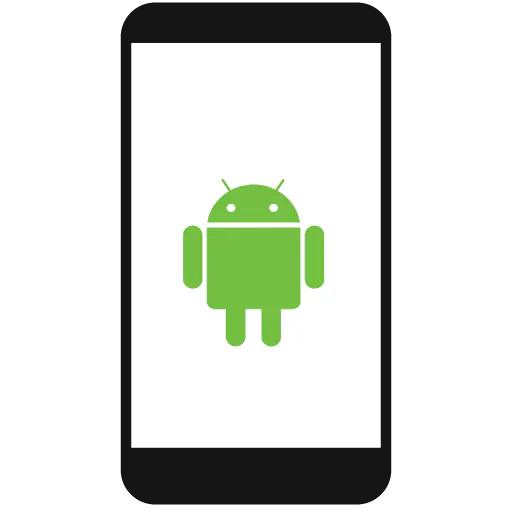Published

# Unlock your door with a knock

Open your door with a special knock using Arduino, Android Phone and 1Sheeld

Full instructions provided30,110## Things used in this project

### Hardware components1Sheeld
×1Android device
×1Arduino UNO & Genuino UNO
×1

## Code

### Untitled file

Arduino
```// Basically the code hear and save the knock and compare it to any other knock to open the door

#include <OneSheeld.h>
int pin1 = 9;
int pin2 = 10;
int pin3 = 11;
int pin4 = 12;
int led4 = 13;
int counter = 0;
int sec = 0;
int hear = {};
int saved = {};
int flagc = 0;
int flags = 0;
int flagf = 0;
int i = 0;
int ii = 0;

void setup()
{
OneSheeld.begin();
pinMode(pin1,OUTPUT);
pinMode(pin2,OUTPUT);
pinMode(pin3,OUTPUT);
pinMode(pin4,OUTPUT);
pinMode(led4,OUTPUT);
}
void loop()
{
{
set();
}
{
record();
}
{
check();
}
else {
flagf = 0;
digitalWrite(pin1,LOW);
digitalWrite(pin2,LOW);
digitalWrite(pin3,LOW);
digitalWrite(pin4,LOW);
digitalWrite(led4,LOW);
}
}

void clc ()
{
counter = 0;
for (int i=0; i<=20;i++)
{
hear[i] = 0;
saved[i] = 0;
}
i = 0;
ii = 0;
digitalWrite(led4,LOW);
}

void set()
{
if (flagf == 0)
{
clc();
}
if(Mic.getValue() > 80 && flags == 0)
{
digitalWrite(led4,HIGH);
saved[ii] = millis() - sec;
sec= millis();
ii++;
flags = 1;
}
if (Mic.getValue() < 80)
{
digitalWrite(led4,LOW);
flags = 0;
}
flagf = 1;
}

void record ()
{

if(Mic.getValue() > 80 && flagc == 0)
{
digitalWrite(led4,HIGH);
hear[i] = millis() - sec;
sec= millis();
i++;
flagc = 1;
}
if (Mic.getValue() < 80)
{
digitalWrite(led4,LOW);
flagc = 0;
}
}

void check ()
{ if (i == ii)
{
for (int j=1;j<=i;j++)
{
int x = hear[j];
int y =saved[j];
if (x+400 >= y && x-400 <=y )
{
counter++;
}
}
if (counter == i)
{
digitalWrite(pin1,HIGH);
digitalWrite(pin2,HIGH);
digitalWrite(pin3,HIGH);
digitalWrite(pin4,HIGH);
}
}
counter = 0;
for (int i=0; i<=20;i++)
{
hear[i] = 0;
}
i = 0;
}
```

### Codebender

https://codebender.cc/sketch:56684

## Credits

### Ashraf Nabil

8 projects • 89 followers